# The uneasy relationship between deep learning and (classical) statistics

An often-expressed sentiment is that deep learning (and machine learning in general) is “simply statistics,” in the sense that it uses different words to describe the same concepts statisticians have been studying for decades. In the 1990s, Rob Tibshirani wrote the following tongue-in-cheek “glossary”::

Something about this table resonates with me.  In fact, as anyone using Pytorch knows, since Tibshiriani posted this table, many of the terms on the right have found broader use in the machine learning community. (And I do hope that statisticians’ grants and conferences have improved as well…)

But thinking of deep learning purely in terms of statistics misses crucial aspects of its success. A better critique of deep learning is that it uses statistical terms to describe radically different concepts. In meme form, it is the “Princess Bride” meme on the right that is a better critique of deep learning than sandserif’s meme on the left.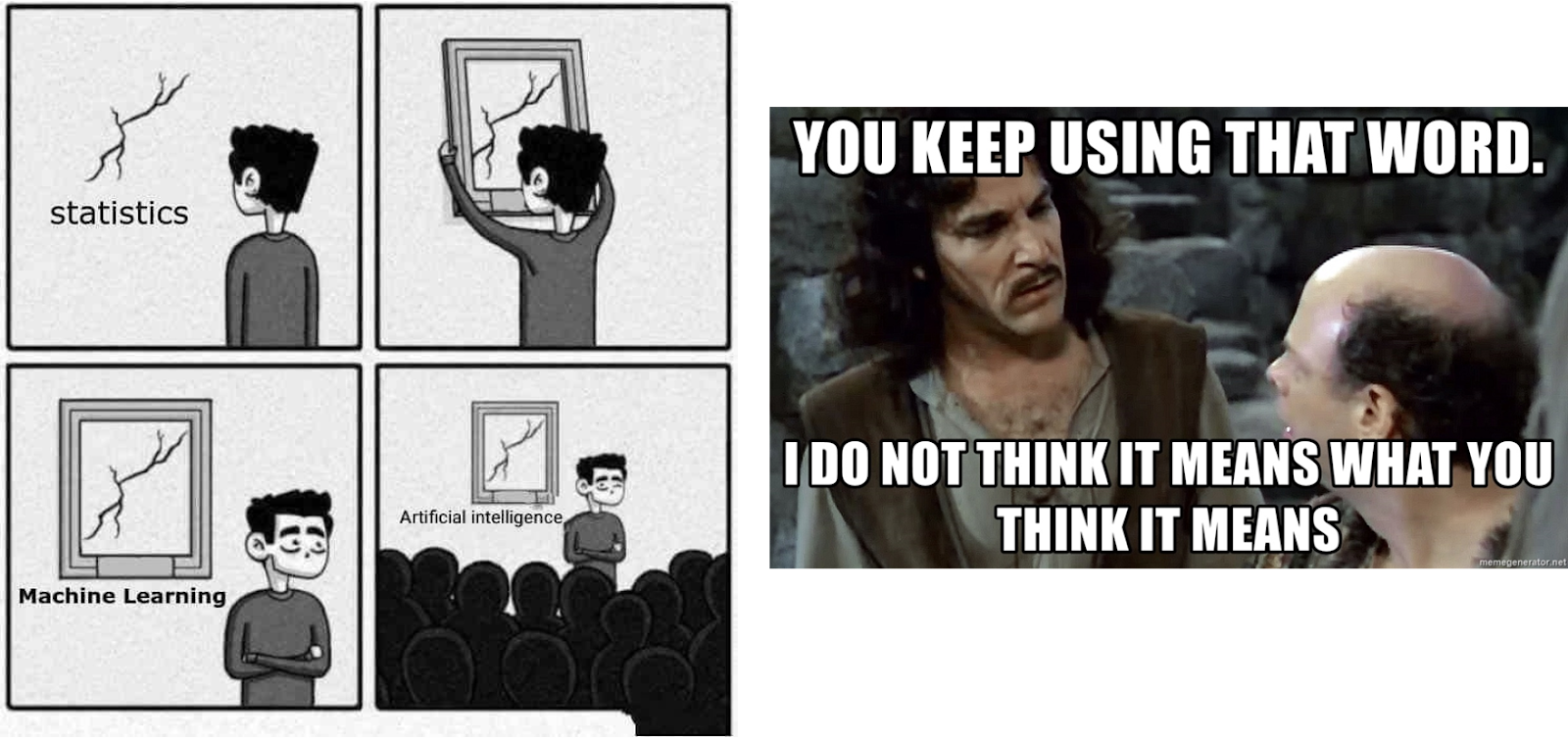Figure: I claim that the right critique of deep learning is not that it uses different words to describe old statistical terms, but rather that it uses these terms to describe a radically different process.

This blog post: organization. In this post, I explain this point of view and why some of the most fundamental aspects of deep learning deviate radically from statistics and even from classical machine learning. In this somewhat long post, I’ll start by talking about the difference between explanation and prediction when fitting models to data. I’ll then discuss two “cartoons” of a learning process: fitting a statistical model using empirical risk minimization and teaching a math skill to a (human) student. I then discuss which one of those processes is a closer match to deep learning. Spoiler: while the math and code of deep learning is nearly identical to the first scenario (fitting a statistical model), I claim that a deeper level, some of deep learning’s most aspects are captured by the “teaching a skill to a student” scenario. I do not claim to have a full theory for deep learning. In fact,I strongly suspect such a theory doesn’t exist. Rather, I believe different aspects of deep learning are best understood from different lenses, and the statistical lens cannot provide the complete picture.

Caveat:  While I contrast deep learning with statistics in this post, I refer to “classical statistics” as it was studied in the past and explained in textbooks. Many statisticians are studying deep learning and going beyond classical methods, analogously to how physicists in the 20th century needed to expand the framework of classical physics. Indeed, the blurring of the lines between computer scientists and statisticians is a modern (and very welcome!) phenomenon that benefits us all.

## 1) Predictions vs. explanations in model fitting.

Scientists have fitted models to observations for thousands of years. For example, as mentioned in my philosophy of science book review post, the Egyptian astronomer Ptolemy came up with an ingenious model for the movement of the planets. Ptolemy’s model was geocentric (with planets rotating around the earth) but had a sequence of “knobs” (concretely, epicycles) that gave it excellent predictive accuracy. In contrast, Copernicus’ initial heliocentric model posited a circular orbit of planets around the sun. It was a simpler model than Ptolemy’s (with fewer “adjustable knobs”) and got the big picture right, but was less accurate in predicting observations. (Copernius later added his own epicycles so he could match Ptolemy’s performance.)

Ptolemy’s and Copernicus’ models were incomparable. If you needed a “black box” for predictions, then Ptolemy’s geocentric model was superior. If you wanted a simple model into which you can “peer inside” and that could be the starting point for a theory to explain the movements of the stars, then Copernicus’ model was better. Indeed, eventually, Kepler refined Copernicus’ models to elliptical orbits and came up with his three laws of planetary movements, which enabled Newton to explain them using the same laws of gravity that apply here on earth. For that, it was crucial that the heliocentric model wasn’t simply a “black box” that provides predictions, but rather was given by simple mathematical equations with few “moving parts.” Over the years, astronomy continued to be an inspiration for developing statistical techniques. Gauss and Legendre (independently) invented least-squares regression around 1800 to predict the orbits of asteroids and other celestial bodies. Cauchy’s 1847 invention of gradient descent was also motivated by astronomical predictions.

In physics, you can (at least sometimes) “have it all” – find the “right” theory that achieves the best predictive accuracy and the best explanation for the data. This is captured by sentiments such as Occam’s Razor, which can be thought of as positing that simplicity, predictive power, and explanatory insights, are all aligned with one another. However, in many other fields, there is a tension between the twin goals of explanation (or, more generally, insight) and prediction. If you simply want to predict observations, then a “black box” could very well be best. On the other hand, if you want to extract insights such as a causal model, general principles, or significant features, then a simpler model that you can understand and interpret might be better. The right choice of model depends on its usage. Consider, for example, a dataset containing genetic expressions and a phenotype (say some disease) for many individuals. If your goal is to predict the chances of an individual getting sick, you want to use the best model for that task, regardless of how complex it is or how many genes it depends on. In contrast, if your goal is to identify a few genes for further investigation in a wet lab, a complicated black box would be of limited use, even if it’s highly accurate.

This point was forcefully made in Leo Breiman’s famous 2001 essay on the two cultures of statistical modeling. The “data modeling culture” focuses on simple generative models that explain the data. In contrast, the “algorithmic modeling culture” is agnostic on how the data is generated and focuses on finding models that predict the data, no matter how complex. Breiman argued that statistics was too dominated by the first culture, and this focus has led to irrelevant theory and questionable scientific conclusions” and prevented statisticians from working on exciting new problems.”

Breiman’s paper was controversial, to say the least. Brad Efron responded to it by saying that, while he agreed with some points, “at first glance, Leo Breiman’s stimulating paper looks like an argument against parsimony and scientific insight, and in favor of black boxes with lots of knobs to twiddle. At second glance, it still looks that way” (see also Kass). In a more recent piece, Efron graciously concedes that “Breiman turned out to be more prescient than me: pure prediction algorithms have seized the statistical limelight in the twenty-first century, developing much along the lines Leo suggested.”

## 2) Classical and modern predictive models.

Machine learning, deep or not, stands firmly in Breiman’s second culture, with a focus on prediction. This culture has a long history.  For example, the following snippets from Duda and Hart’s 1973 textbook and Highleyman’s 1962 paper would be very recognizable to deep learning practitioners today:

Similarly, Highleyman’s handwritten characters dataset and the architecture Chow (1962)  used to fit it  (with ~58% accuracy) would also strike a chord with modern readers, see the Hardt-Recht book and their blog post.

## 3) Why deep learning is different.

In 1992, Geman, Bienenstock, and Doursat wrote a pessimistic article about neural networks, arguing that “current-generation feed-forward neural networks are largely inadequate for difficult problems in machine perception and machine learning”. Specifically, they believed that general-purpose neural networks would not be successful in tackling difficult tasks, and the only way for them to succeed would be via hand-designed features. In their words: “important properties must be built-in or “hard-wired” … not learned in any statistically meaningful way.” In hindsight (which is always 20/20), Geman et al. were completely wrong (if anything, modern architectures such as transformers are even more general than the convolutional networks that existed at the time), but it is interesting to understand why they were wrong.

I believe that the reason is that deep learning is genuinely different from other learning methods. A priori, it seems that deep learning is just one more predictive model, like nearest neighbors or random forests. It may have more “knobs,” but that seems to be a quantitative rather than qualitative difference. However, in the words of P.W. Andreson, “more is different.”    In Physics, we often need a completely different theory once scale changes by several orders of magnitude, and the same holds in deep learning. The processes that underlie deep learning vs. classical models (parametric or not) are radically different, even if the equations (and Python code) look identical at a high level.

To clarify this point, let’s consider two very different learning processes: fitting a statistical model and teaching math to a student.

### Scenario A: Fitting a statistical model

Classically, fitting a statistical model to data corresponds to the following:

1. We observe some data$X,y$. (Think of$X$ as an$n\times p$ matrix and$y$ as an$n$ dimensional vector; think of the data as coming from a structure and noise model: each coordinate$y_i$ is obtained as$f_0(x_i)+e_i$ where$e_i$ is the corresponding noise , using additive noise for simplicity, and$f_0$ as the “ground truth.”)
2. We fit a model$\hat{f}$ to the data by running some optimization algorithm to minimize an empirical risk of$\hat{f}$. That is, we use optimization to (try to) find$\hat{f}$ that minimizes a quantity$L(\hat{f}) + R(\hat{f})$ where$L(\cdot)$ is a loss term (capturing how close$\hat{f}(X)$ is to$y$) and$R(\hat{f})$ is an optional regularization term (attempting to bias$\hat{f}$ toward simpler models under some measure).
3. Our hope is that our model will have good population loss, in the sense that the generalization error/loss$\mathbb{E} [L(\hat{f}(x),y)]$ is small (where this expectation is taken over the total population from which our data was drawn).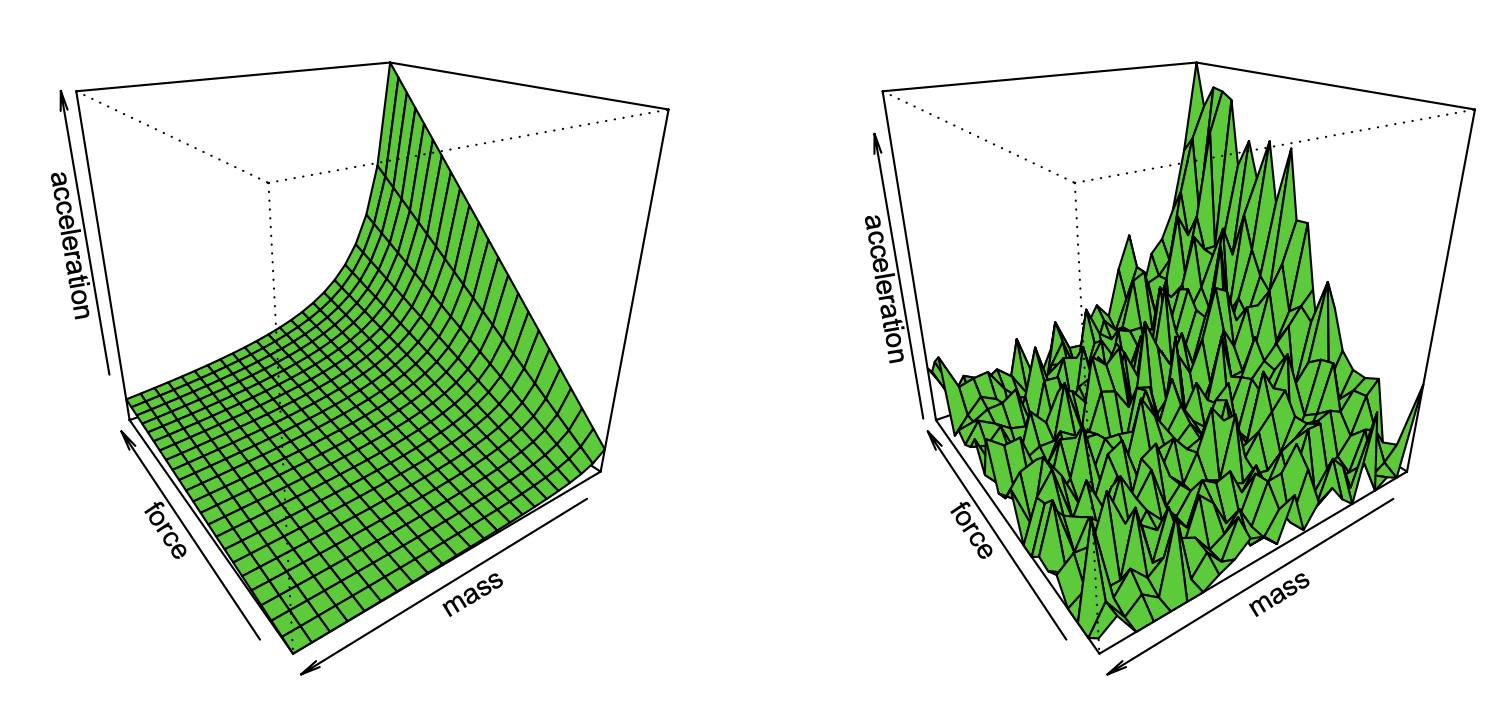Figure: Effron’s cartoon of recovering Newton’s first law from noisy observations.

This very general paradigm captures many settings, including least-squares linear regression, nearest neighbors,  neural network training, and more. In the classical statistical setup, we expect to observe the following:

Bias/variance tradeoff: Let$\mathcal{F}$ be the set of models that we optimize over. (If we are in the non-convex setting and/or have a regularizer term, we can let$\mathcal{F}$ be the set of such models that can be achieved by the algorithm with non-negligible probability, taking the effects of algorithm choice and regularizer into account.)  The bias of$\mathcal{F}$ is the best approximation to the ground truth that can be achieved by an element$\hat{f} \in \mathcal{F}$. The larger the class$\mathcal{F}$, the smaller the bias, and it can be zero if$f_0 \in \mathcal{F}$. However, the larger the class$\mathcal{F}$, the more samples we would need to narrow down its members and hence the more variance in the model that the algorithm outputs. The overall generalization error is the sum of the bias term and the contribution from the variance. Hence statistical learning typically displays a bias/variance tradeoff, with a “goldilocks choice” of the right model complexity that minimizes the overall error.  Indeed, Geman et al. justified their pessimism on neural networks by saying that “the fundamental limitations resulting from the bias-variance dilemma apply to all nonparametric inference models, including neural networks.”

More is not always better. In statistical learning, getting more features or data does not necessarily improve performance. For example, learning from data that contains many irrelevant features is more challenging. Similarly, learning from a mixture model, in which data comes from one of two distributions (e.g.,$y=f_0(x)$ and$y=f_1(x)$), is harder than learning each distribution independently.

Diminishing returns. In many settings, the number of data points needed to reduce the prediction noise to a level of$\epsilon$ scales as$k/\epsilon^2$ for some parameter$k$. In such cases, it takes about$k$ samples to “get off the ground” but once we do so we face a regime of diminishing returns, whereby if it took$n$ points to achieve (say) 90% accuracy, it will take roughly an additional$3n$ points to increase accuracy to 95%. In general, as we increase our resources (whether data, model complexity, or computation) we expect to capture finer and finer distinctions rather than unlocking qualitatively new capabilities.

Strong dependence on loss, data. When fitting a model to high-dimensional data, small details can make a big difference. Statisticians know that choices such as an L1 or L2 regularizer matter, not to mention using completely different datasets (e.g., Wikipedia vs. Reddit). High-dimensional optimizers of different quantities will be very different from one another.

No natural “difficulty” of data points (at least in some settings). Traditionally, we think of data points as sampled independently from some distribution. Though points closer to the decision boundary could be harder to classify, given the concentration-of-measure phenomena in high dimensions, we expect that most points would be of similar distance. Thus at least in classical data distributions, we don’t expect points to vary greatly in their difficulty level. However, mixture models can display such variance in difficulty level, and hence, unlike the other issues above, such variance would not be terribly surprising in the statistical setting.

### Scenario B: Learning math

In contrast to the above, consider the setting of teaching a student some particular topic in mathematics (e.g., computing derivatives), by giving them general instructions, as well as exercises to work through. This is not a formally defined setting, but let’s consider some of its qualitative features: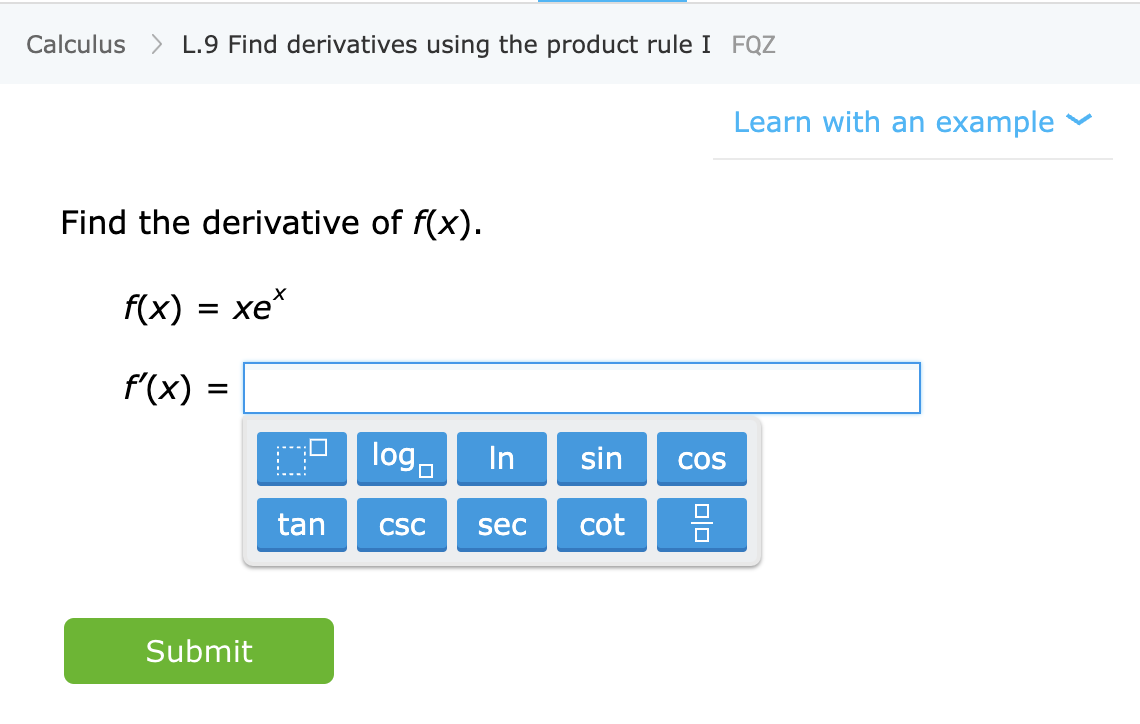Figure: An exercise to learn a particular math skill from the IXL website.

Learning a skill, rather than approximating a distribution. In this setting, the student learns a skill rather than an estimator/predictor for some quantity. While defining “skill” is not a trivial task (and not one we’ll undertake in this blog post), it is a qualitatively different object. In particular, even if the function mapping exercises to solutions cannot be used as a “black box” to solve some related task X, we believe that the internal representations that the student develops while working through these problems can still be useful for X.

More is better. Generally, students that do more problems and problems of different types achieve better performance. A “mixture model” – doing some calculus problems and some algebra problems – does not hurt the student in their calculus performance and in fact, could only help.

“Grokking” or unlocking capabilities, moving to automatic representations. While at some point there are diminishing returns also when solving problems, students do seem to undergo several phases. There is a stage in which doing some problems helps a concept “click” and unlocks new capabilities. Also, as students repeat problems of a specific type, they seem to move their facilities and representations of these problems to a lower level, enabling certain automaticity with them that they didn’t have before.

Performance is partially independent of the loss and data. There is more than one way to teach mathematical concepts. Students who study with different books, educational approaches, or grading systems can eventually learn the same material and (as far as we can tell) similar internal representations of it.

Some problems are harder than others. In math exercises, we often see a strong correlation between how different students solve the same problem. There does seem to be an inherent difficulty level for a problem and a natural progression of difficulty that is optimal for learning. Indeed this is precisely what is being done by platforms such as IXL.

## 4) Is deep learning more like statistical estimation or a student learning a skill?

So, which of the above two metaphors more appropriately captures modern deep learning, and specifically the reasons why it is so successful? Statistical model fitting seems to correspond well to the math and the code. Indeed the canonical Pytorch training loop trains deep networks through empirical risk minimization as described above:

However, on a deeper level, the relation between the two settings is not as clear. For concreteness, let us fix a particular learning task. Consider a classification algorithm that is trained using the method of “self-supervised learning + a linear probe” (what we called Self-Supervised + Simple or SSS in our paper with Bansal and Kaplun). Concretely, the algorithm is trained as follows:

1. Suppose that the data is a sequence$\{ (x_i,y_i) \}_{i=1..n}$ where$x_i \in \mathbb{R}^p$ is some datapoint (say an image for concreteness) and$y_i$ is a label.
2. We first find a deep neural network implementing representation function$\hat{r}:\mathbb{R}^p \rightarrow \mathbb{R}^d$. This function is trained only using the datapoints$\{ x_1,\ldots, x_n \}$ and not using the labels by minimizing some type of a self-supervised loss function. Example of such loss functions are reconstruction or in-painting (recovering some part of the input$x$ from another) or contrastive learning (finding$\hat{r}$ such that$\parallel \hat{r}(x)-\hat{r}(x') \parallel$ is significantly smaller when$x,x'$ are augmentations of the same datapoint than when they are two random points).
3. We then use the full labeled data$\{ (x_i,y_i )$ to fit a linear classifier$\hat{\ell}:\mathbb{R}^d \to \mathbb{R}^c$ (where$c$ is the number of classes) that minimizes the cross-entropy loss. Our final classifier is the map$x \mapsto \mathrm{arg}\max \hat{\ell}(\hat{r}(x))$.

Step 3 merely fits a linear classifier and so the “magic” happens in step 2 (self-supervised learning of a deep network). Some of the properties we see in self-supervised learning include:

Learning a skill rather than approximating a function. Self-supervised learning is not about approximating a function but rather learning representations that could be used in a variety of downstream tasks. For example, this is the dominant paradigm in natural language processing. Whether the downstream task is obtained through linear probe, fine tuning, or prompting is of secondary importance.

More is better. In self-supervised learning, representation quality improves with data quantity. We don’t suffer from mixing in several sources: in fact, the more diverse the data is, the better.

Unlocking capabilities. We have seen time and again discontinuous improvements in deep learning models as we scale resources (data, compute, model size). This has also been demonstrated in some synthetic settings.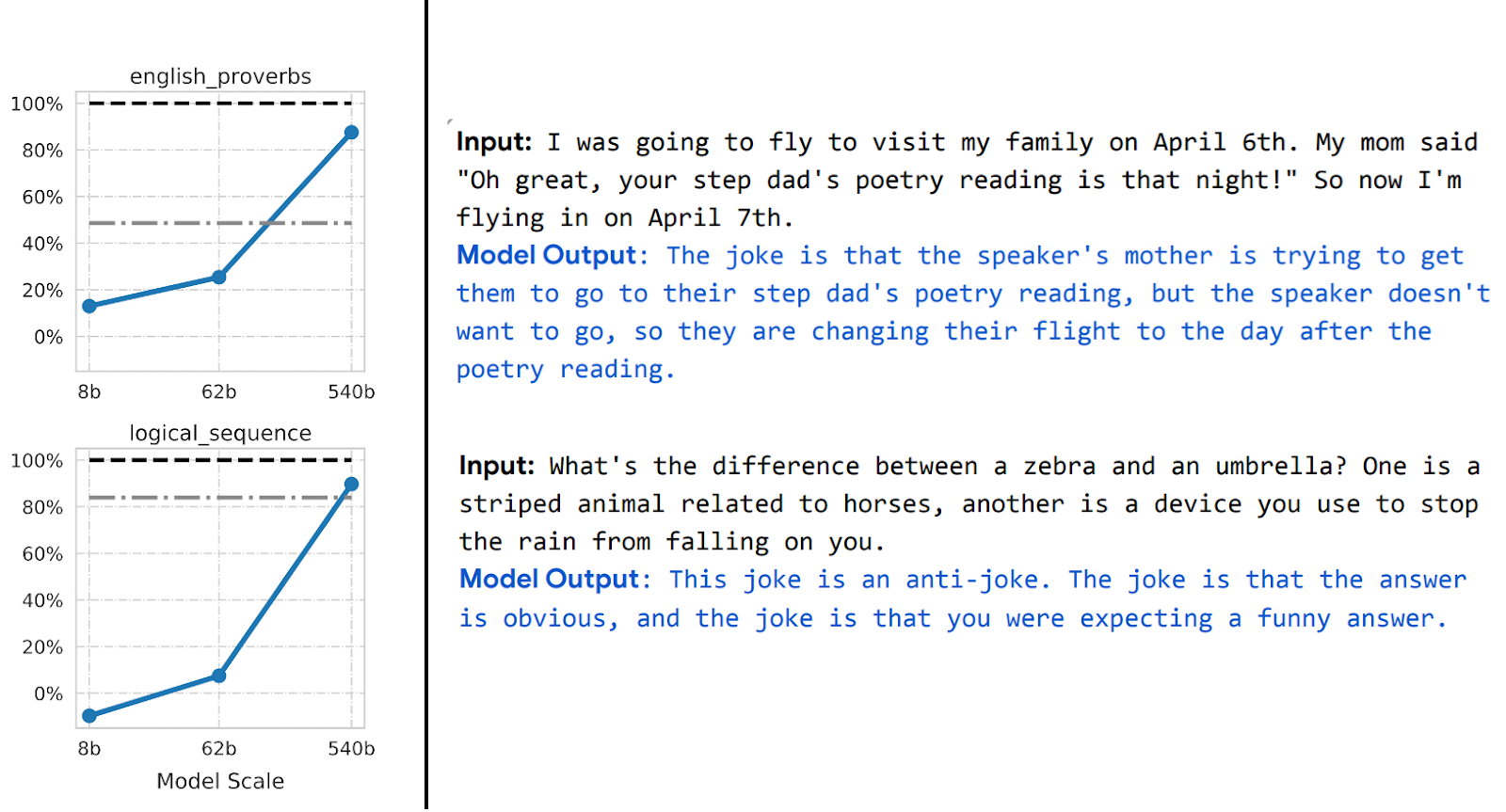Figure: The PaLM model displays some discontinuous improvements in some benchmarks as model size increases (with caveat of only three sizes in these plots), with some surprising capabilities unlocked such as explaining jokes.

Performance is largely independent of loss or data. There is more than one self-supervised loss. Several contrastive and reconstruction losses have been used for images. For language models, we sometimes use one-sided reconstruction (predict next token) and sometimes masked models whose goal is to predict a masked input from both the left and right token. We can also use slightly different datasets. These can make differences in efficiency, but as long as we make “reasonable” choices, typically raw resources are more significant predictors of performance than the particular loss or dataset used.

Some instances are harder than others. This point is not specific to self-supervised learners. It does seem that data points have some inherent “difficulty level”. Indeed, we have several pieces of empirical evidence for the notion that different learning algorithms have a different “skill level” and different points have a different “difficulty level” (with the probability of classifier$f$ classifying point$x$ correctly being monotonically increasing with$f$’s skill and monotonically decreasing with$x$’s difficulty). The “skill vs. difficulty” paradigm is the cleanest explanation for the “accuracy on the line” phenomenon uncovered by Recht et al and  Miller et al. Our paper with Kaplun, Ghosh, Garg, and Nakkiran also shows how different inputs in datasets have an inherent “difficulty profile” that seems to be generally robust with respect to different model families.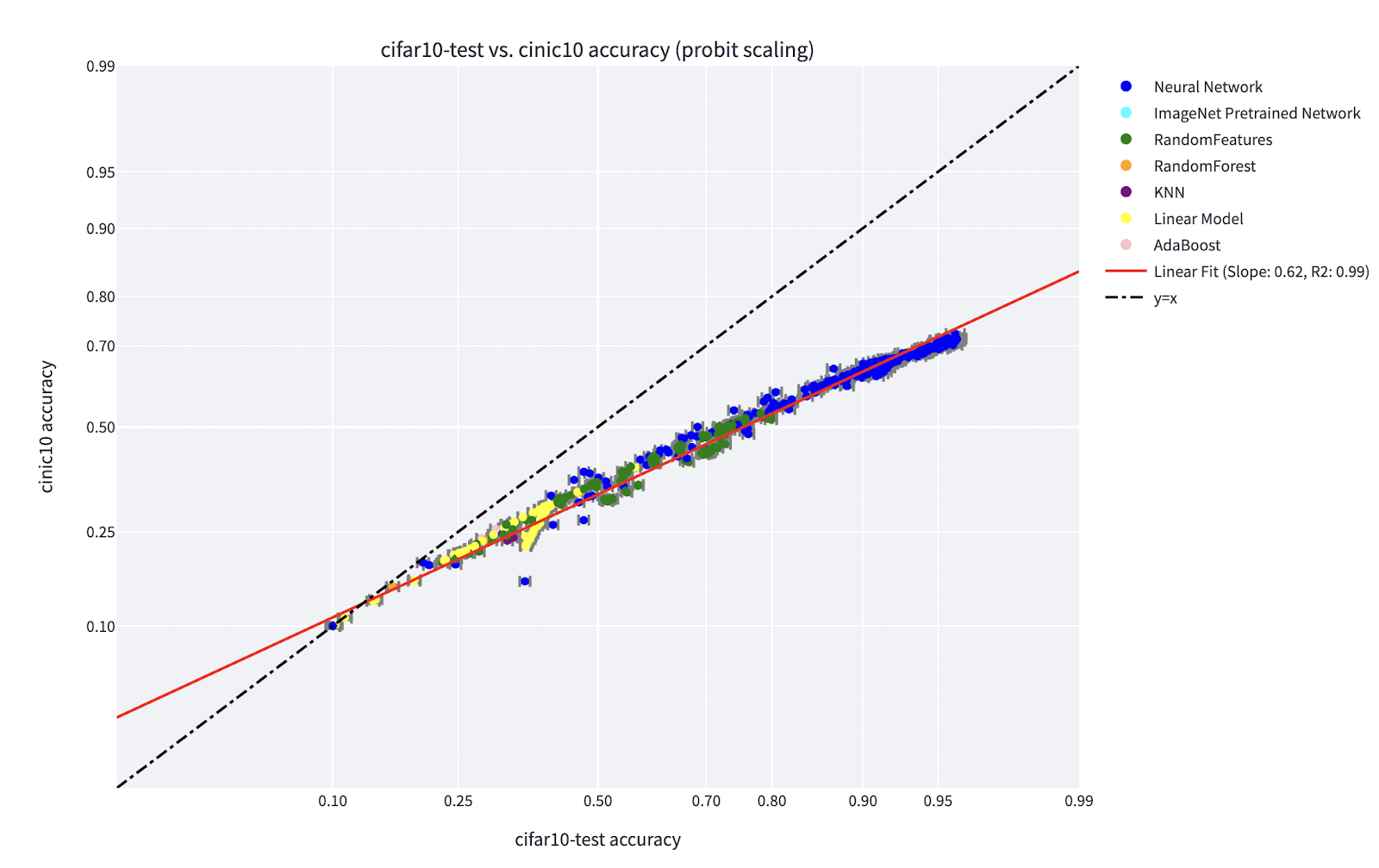Figure: Miller et al’s graph showing accuracy on the line phenomena for classifiers trained on CIFAR-10 and tested on CINIC-10.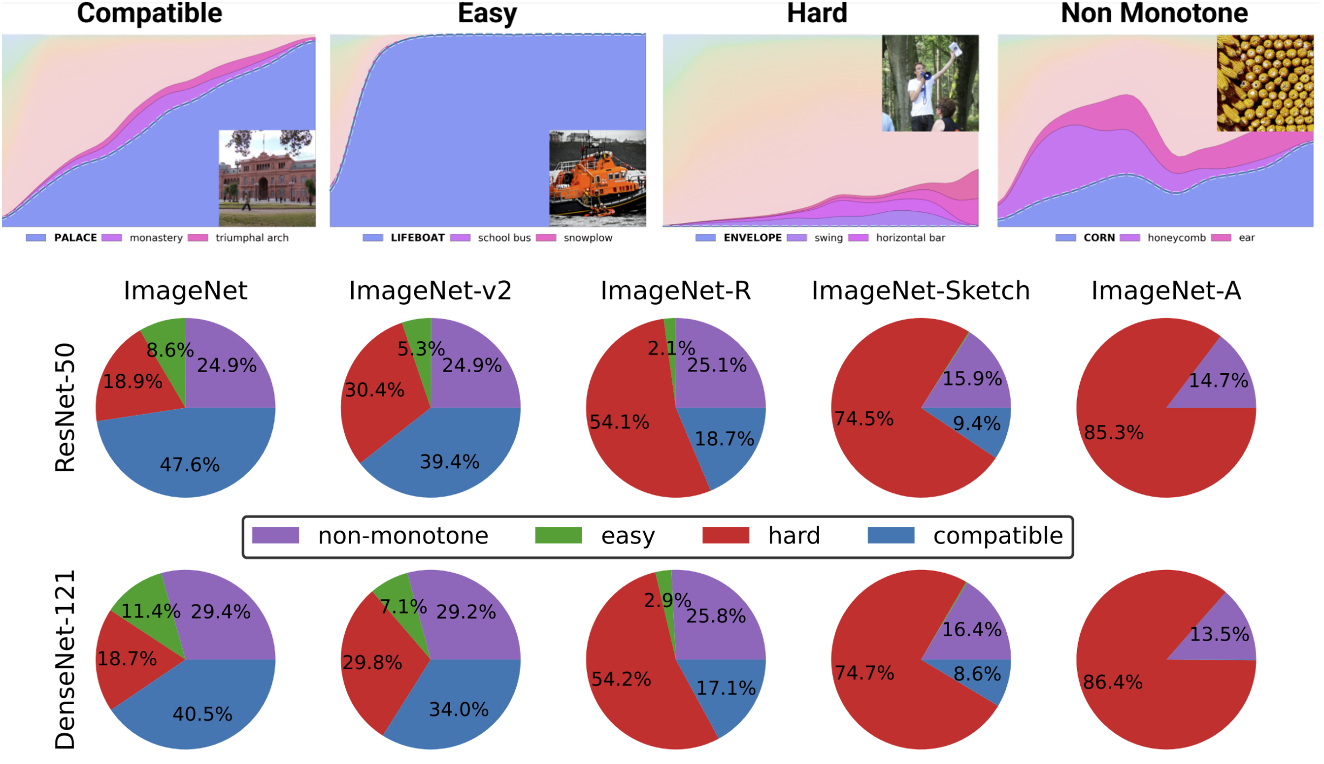Figure: Deconstruction of datasets into points from Kaplun and Ghosh et al of different “difficulty profiles” for increasingly more resourced classifiers. The graphs at the top describe the different softmax probabilities for most likely classes as a function of the global accuracy of a classifier from a certain family indexed by training time. The pie charts at the bottom show the decomposition of different datasets into points of the varying types. Note that this decomposition is similar with respect to different neural architectures.

Training as teaching. Training of modern large models seems much more like teaching a student than fitting a model to data, complete with “taking breaks” or trying different approaches when the student doesn’t get it or seems tired (training diverges). The training logbook of Meta’s large model is instructive- aside from issues with hardware, we can see interventions such as switching different optimization algorithms in the middle of training and even considering “hot swapping” the activation functions (GELU to RELU). The latter doesn’t make much sense if you think of model training as fitting data as opposed to learning representations.

## 4.1) But what about supervised learning?

Up to this point, we only discussed self-supervised learning, but the canonical example of deep learning- the one you teach first in a course- is still supervised learning. After all, deep learning’s “ImageNet moment” came with, well, ImageNet. Does anything we said above still apply to this setting?

First, the emergence of supervised large-scale deep learning is to some extent a historical accident, aided by the availability of large high quality labeled datasets (i.e. ImageNet). One could imagine an alternative history in which deep learning first started showing breakthrough advances in Natural Language Processing via unsupervised learning, and only later transported into vision and supervised learning.

Second,  we have some evidence that even though they use radically different loss functions, supervised and self-supervised learning behave similarly “under the hood.” Both often achieve the same performance, and in work with Bansal and Nakkiran, we showed that they also learn similar internal representations. Concretely, for every$k \in \{1..d-1\}$, one can “stitch together” the first$k$ layers of a depth$d$ model that was trained via self-supervision with the last$d-k$ layers of a supervised model with little loss in performance.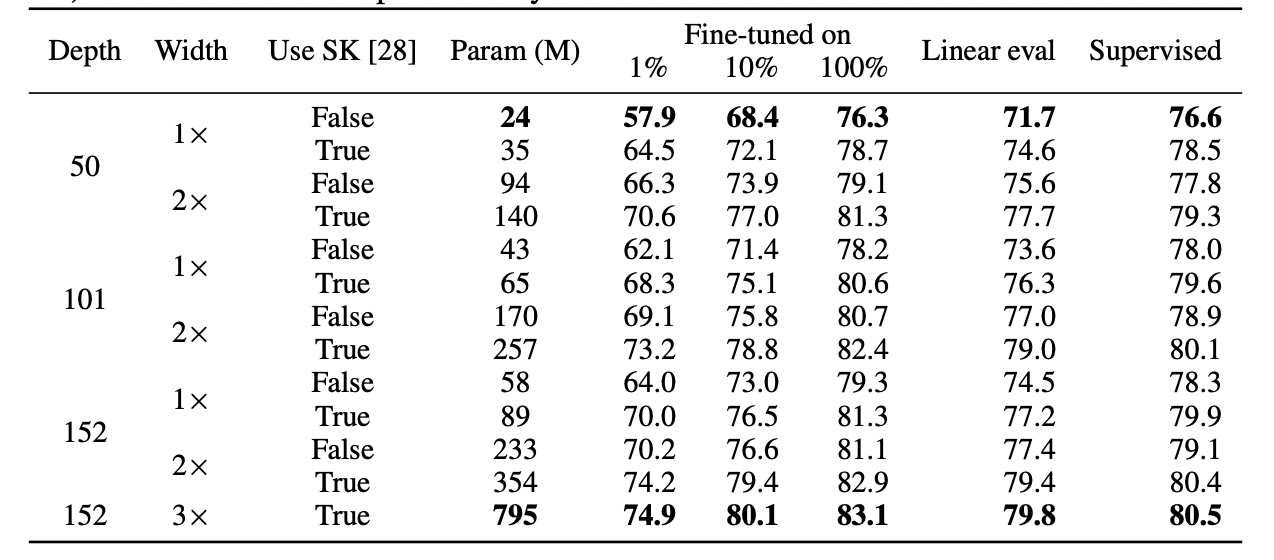Figure: Table from SimCLR v2 paper (Chen et al). Note the general similarity in performance between supervised learning, fine-tuned (100%) self-supervised, and self-supervised + linear probe.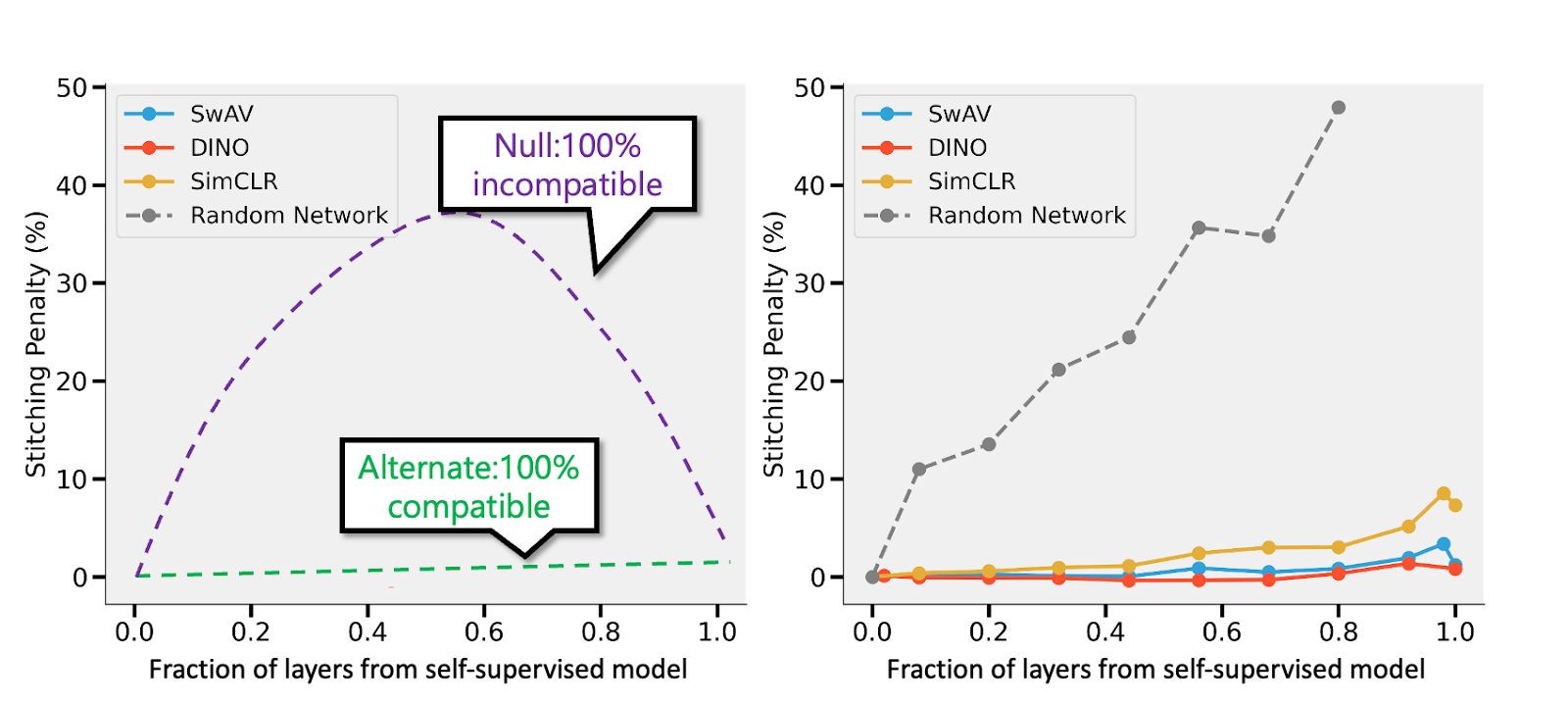Figure: Stitching a self supervised and a supervised model from Bansal et al. Left: If the self-supervised is (say) less accurate than the supervised model, then fully compatible representations would result in a stitching penalty of when fraction of layers come from the self-supervised model. If the models are completely incompatible then we expect accuracy to drop sharply as we stitch more models. Right: Actual results for stitching different self-supervised models. We also stitch a random network as a “sanity check”.

The advantage of self-supervised + simple models is that they can separate out the aspects of feature learning or “deep learning magic” (done by the deep representation function) from the statistical model fitting (done by the linear or other “simple” classifier on top of this representation).

Finally, while this is more speculative, the fact that often “meta learning” seems to amount to learning representations (see Raghu et al. and Mandi et. al.)  can be considered as another piece of evidence that this is much of what’s going on, regardless of the objective that the model ostensibly optimizes.

## 4.2) What about over parameterization?

The reader may have noticed that I skipped over what is considered the canonical example of the disparity between the model of statistical learning and deep learning in practice: the absence of a “bias-variance tradeoff” (see Belkin et al.’s double descent) and the ability of over-parameterized models to generalize well.

There are two reasons I do not focus on this aspect. First, if supervised learning really does correspond to self-supervised + simple learning “under the hood” then that may explain its generalization ability.  Second, I think that over parameterization is not crucial to deep learning’s success. Deep networks are special not because they are big compared to the number of samples but because they are big in absolute terms. Indeed, typically in unsupervised / self-supervised learning models are not over parameterized. Even for the very large language models, their datasets are larger still. This does not make their performance any less mysterious.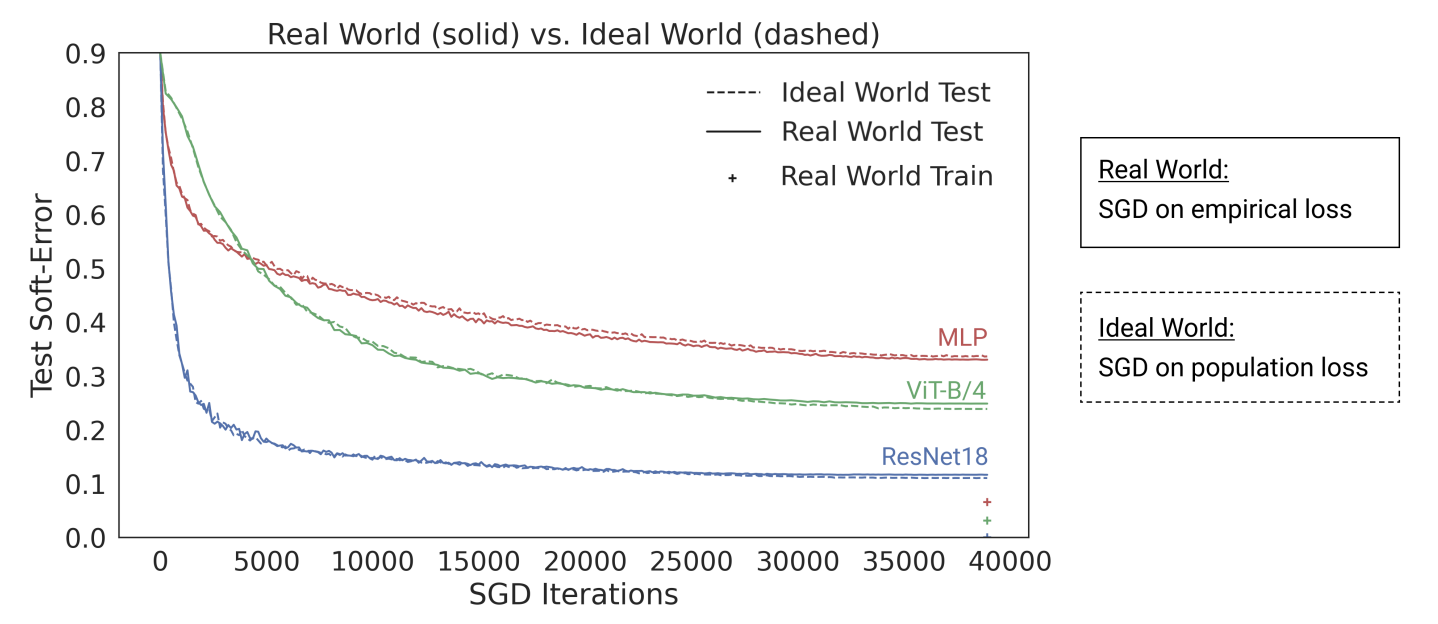Figure: Nakkiran-Neyshabur-Sadghi’s “deep bootstrap” paper demonstrates that modern architectures behave similarly in the “over parameterized” or “under sampled” regime (where models train for multiple epochs on limited data until over fitting: “real world” in the above figure) and “under parameterized” or “online” regime (where models train for a single epoch, seeing each sample only once: “ideal world” in the above figure).

## Summary

Statistical learning certainly plays a role in deep learning. However, despite using similar terms and code, thinking of deep learning as simply fitting a model with more knobs than classical models misses a lot of what is essential to its success. The human student metaphor is hardly perfect either. Like biological evolution, even though deep learning consists of many repeated applications of the same rule – gradient descent on empirical loss- it gives rise to highly complex outcomes. It seems that at different times different components of networks learn different things, including, representation learning, prediction fitting, implicit regularization, and pure noise. We are still searching for the right lens by which to ask questions about deep learning, let alone answer them.

Acknowledgments: Thanks to Lucas Janson and Preetum Nakkiran for comments on early versions of this blog post.

## 2 thoughts on “The uneasy relationship between deep learning and (classical) statistics”

1.Alex Wei says:

Very inspiring article!